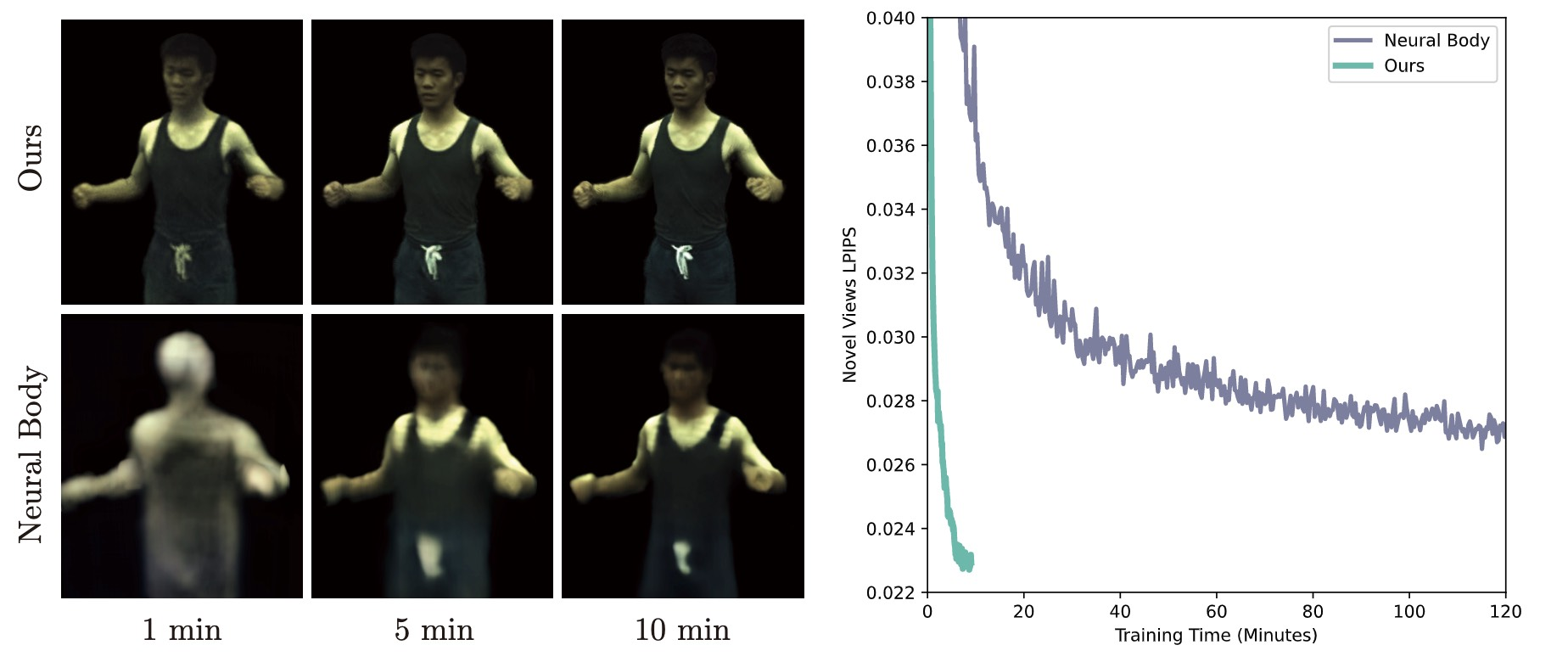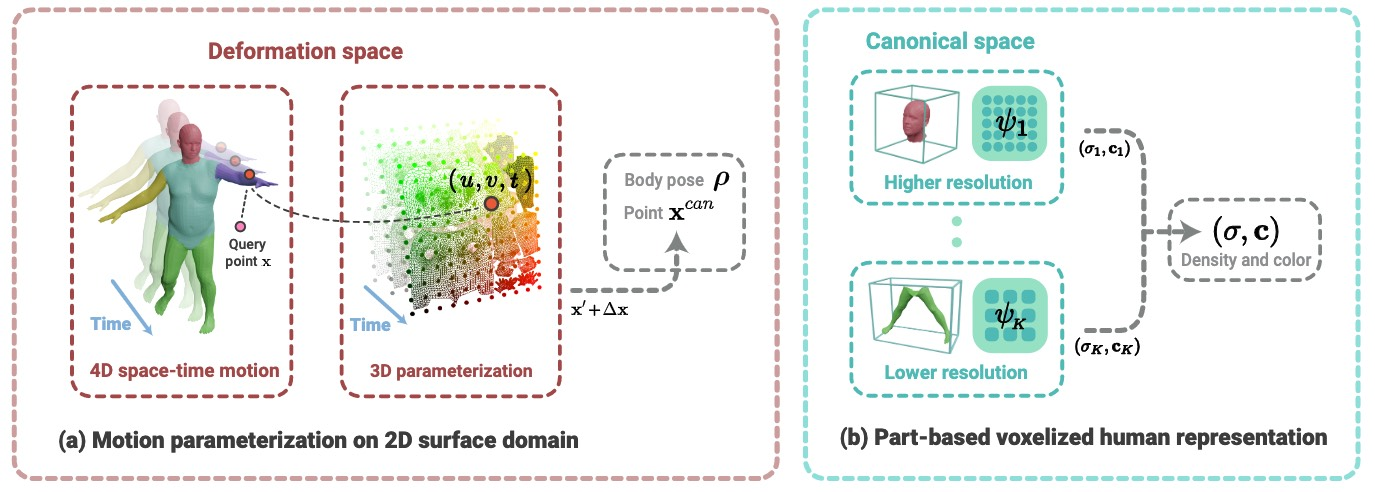## Learning Neural Volumetric Representations of Dynamic Humans in Minutes CVPR 2023

(* equal contribution)
State Key Laboratory of CAD&CG, Zhejiang UniversityComparison of optimization speed between our method and baseline.

### Abstract

This paper addresses the challenge of quickly reconstructing free-viewpoint videos of dynamic humans from sparse multi-view videos. Some recent works represent the dynamic human as a canonical neural radiance field (NeRF) and a motion field, which are learned from videos through differentiable rendering. They generally require a lengthy optimization process. Other generalization methods leverage learned prior from datasets and reduce the optimization time by only finetuning on new scenes at the cost of visual fidelity. In this paper, we propose a novel method for creating viewpoint-free human performance synthesis from sparse view videos in minutes with competitive visual quality. Specifically, we leverage the human body prior to define a novel part-based voxelized NeRF representation, which distributes the representational power of the canonical human model efficiently. Furthermore, we propose a novel dimensionality reduction 2D motion parameterization scheme to increase the convergence rate of the human deformation field. Experiments demonstrate that our approach can be trained 100 times faster than prior per-scene optimization methods while being competitive in the rendering quality. We show that given a 512x512 video capturing a human performer of 100 frames, our model typically takes about 5 minutes for training to produce photorealistic free-viewpoint videos on a single RTX 3090 GPU. The code will be released for reproducibility.

### MethodGiven a query point $\mathbf{x}$ at frame $t$, we find its nearest surface point on each human part of the SMPL mesh, which gives the blend weight $\mathbf{w}_k$ and the UV coordinate $(u_k, v_k)$. Consider the $k$-th part. The motion field consists of an inverse LBS module and a residual deformation module. (a) The inverse LBS module takes body pose $\boldsymbol{\rho}$, blend weight $\mathbf{w}_k$, and query point $\mathbf{x}$ as input and output the transformed point $\mathbf{x}'$. The residual deformation module applies the multiresolution hash encoding (MHE) to $(u_k, v_k, t)$ and uses an MLP network to regress the residual translation $\Delta \mathbf{x}$, which is added to $\mathbf{x}'$ to obtain the canonical point $\mathbf{x}^{\text{can}}$. (b) We then feed $\mathbf{x}^{\text{can}}$ to networks of $k$-th human part to predict the density $\sigma_k$ and color $\mathbf{c}_k$. With $\{(\sigma_k, \mathbf{c}_k)\}_{k=1}^K$, we select the one with the biggest density as the density and color of the query point.

### Qualitative Comparison

Ours
(trained for ~5 min)

Neural Body
(trained for ~10 hours)

Animatable NeRF
(trained for ~10 hours)

### Citation

The website template was borrowed from Michaël Gharbi and Jon Barron. Last updated: 02/24/2023.